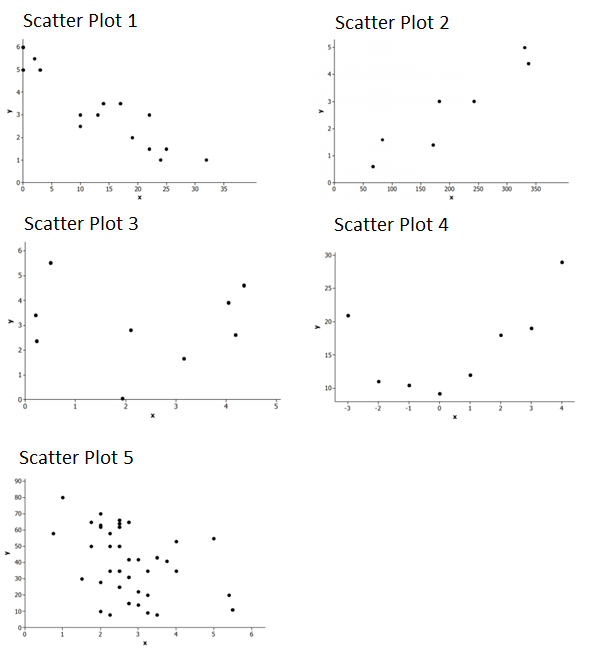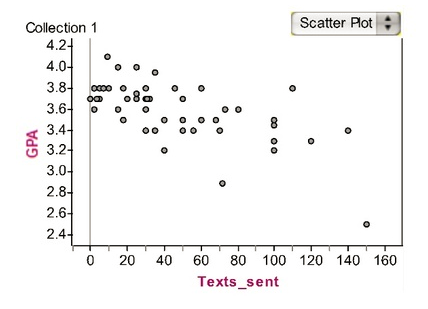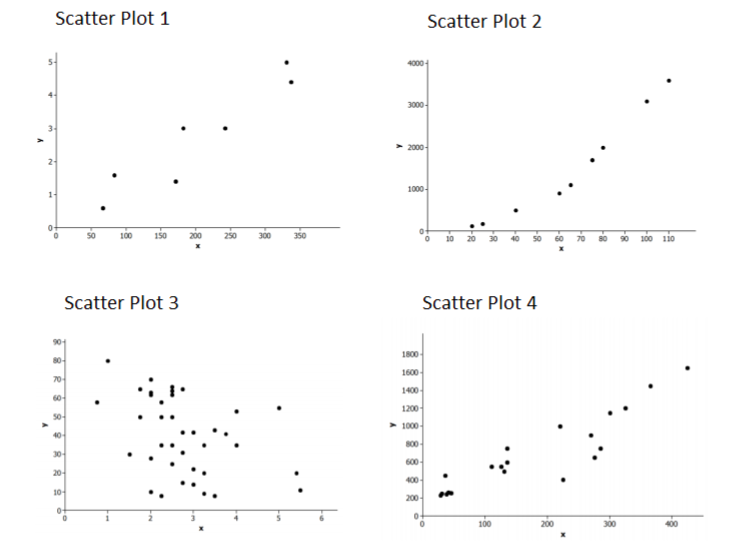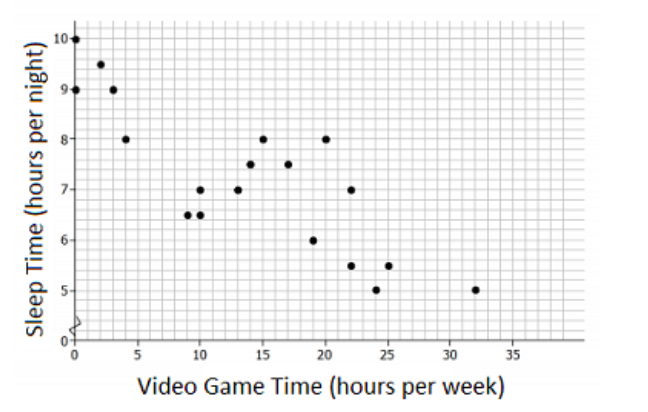# Bivariate Data

## Objective

Identify and describe associations in scatter plots including linear/nonlinear associations, positive/negative associations, clusters, and outliers.

## Common Core Standards

### Core Standards

?

• 8.SP.A.1 — Construct and interpret scatter plots for bivariate measurement data to investigate patterns of association between two quantities. Describe patterns such as clustering, outliers, positive or negative association, linear association, and nonlinear association.

?

• 8.F.A.3

• 8.F.B.4

• 8.F.B.5

• 6.SP.A.2

## Criteria for Success

?

1. Identify linear vs. nonlinear associations in scatter plots.
2. Identify and describe positive associations in scatter plots, where as $x$ increases, then $y$ tends to increase as well.
3. Identify and describe negative associations in scatter plots, where as $x$ increases, then $y$ tends to decrease.
4. Identify and explain the significance of outliers and clusters in scatter plots.

## Tips for Teachers

?

Students re-engage in their work on linear relationships as they analyze scatter plots for possible linear associations. They recall positive and negative slopes in the coordinate plane and use this understanding to describe behaviors of one variable in relation to the other.

#### Fishtank Plus

• Problem Set
• Student Handout Editor
• Vocabulary Package

## Anchor Problems

?

### Problem 1

For the following five scatter plots, answer the following questions:

1. Does there appear to be a relationship between $x$ and $y$?
2. If there is a relationship, does it appear to be linear?
3. If the relationship appears to be linear, is it a positive or negative linear relationship?
4. If applicable, circle the correct words in this sentence: There is a (positive/negative) association between $x$ and $y$ because as $x$ increases, then $y$ tends to (increase/decrease).#### References

EngageNY Mathematics Grade 8 Mathematics > Module 6 > Topic B > Lesson 7Exercise 1-5

Grade 8 Mathematics > Module 6 > Topic B > Lesson 7 of the New York State Common Core Mathematics Curriculum from EngageNY and Great Minds. © 2015 Great Minds. Licensed by EngageNY of the New York State Education Department under the CC BY-NC-SA 3.0 US license. Accessed Dec. 2, 2016, 5:15 p.m..

Modified by The Match Foundation, Inc.

### Problem 2

Medhavi suspects that there is a relationship between the number of text messages high school students send and their academic achievement. To explore this, she asks each student in a random sample of 52 students from her school how many text messages he or she sent yesterday and what his or her grade point average (GPA) was during the most recent marking period. The data are summarized in the scatter plot of number of text messages sent versus GPA shown below.1. Describe the relationship between the number of text messages sent and GPA.
2. Are there any deviations from the pattern? Explain what you see.
3. Are there any clusters of data? What do they mean in context?

#### References

Illustrative Mathematics Texting and Grades I

Texting and Grades I, accessed on April 9, 2018, 2:36 p.m., is licensed by Illustrative Mathematics under either the CC BY 4.0 or CC BY-NC-SA 4.0. For further information, contact Illustrative Mathematics.

Modified by The Match Foundation, Inc.

## Problem Set

?The following resources include problems and activities aligned to the objective of the lesson that can be used to create your own problem set.

• Examples where students sketch their own examples of different relationships; for example, sketch a scatter plot of two variables with a moderate linear relationship and 2 outliers

?

### Problem 1

Which of the following scatter plots shows a negative linear relationship? Explain how you know.#### References

EngageNY Mathematics Grade 8 Mathematics > Module 6 > Topic B > Lesson 7Exit Ticket, Question #1

Grade 8 Mathematics > Module 6 > Topic B > Lesson 7 of the New York State Common Core Mathematics Curriculum from EngageNY and Great Minds. © 2015 Great Minds. Licensed by EngageNY of the New York State Education Department under the CC BY-NC-SA 3.0 US license. Accessed Dec. 2, 2016, 5:15 p.m..

### Problem 2

The scatter plot below was constructed using data from eighth-grade students on number of hours playing video games per week ($x$) and number of hours of sleep per night ($y$).Which of the following statements are true about the scatter plot shown? Select all that apply.

1. There is negative linear relationship between the number of hours per week playing video games and the number of hours per night sleeping.
2. There is a positive linear relationship between the number of hours per week playing video games and the number of hours per night sleeping.
3. As video game time increases, the number of hours of sleep tends to increase as well.
4. As video game time decreases, the number of hours of sleep tends to increase.
5. There is a cluster represented at 32 hours of video game time.

#### References

EngageNY Mathematics Grade 8 Mathematics > Module 6 > Topic B > Lesson 7Exit Ticket, Question #2

Grade 8 Mathematics > Module 6 > Topic B > Lesson 7 of the New York State Common Core Mathematics Curriculum from EngageNY and Great Minds. © 2015 Great Minds. Licensed by EngageNY of the New York State Education Department under the CC BY-NC-SA 3.0 US license. Accessed Dec. 2, 2016, 5:15 p.m..

Modified by The Match Foundation, Inc.

?